International
Tables for
Crystallography
Volume F
Crystallography of biological macromolecules
Edited by M. G. Rossmann and E. Arnold

International Tables for Crystallography (2006). Vol. F, ch. 11.3, pp. 221-222   | 1 | 2 |

## Section 11.3.3. Integration

W. Kabscha*

aMax-Planck-Institut für medizinische Forschung, Abteilung Biophysik, Jahnstrasse 29, 69120 Heidelberg, Germany
Correspondence e-mail: kabsch@mpimf-heidelberg.mpg.de

### 11.3.3. Integration

| top | pdf |

A fundamental requirement for a general integration method is that it should distinguish carefully between signal and background points within its integration domain. For weak reflections, this distinction cannot be made reliably because of the errors superimposed on the signal. The problem can be solved, however, provided that both weak and strong reflections share the same profile shape – an assumption that has been adopted by most data-processing packages.

The intensity distribution of a reflection can be modelled analytically or derived from the observed profiles of neighbouring strong spots. For the rotation method, the profile shape depends strongly on the specific path of the reflection through the Ewald sphere and on variations in the angle of incidence of the diffracted beam on a flat detector. These geometrical distortions can be eliminated by mapping the reflections onto the coordinate system defined in Section 11.3.2.3, which simplifies the task of modelling the expected intensity distribution as all reflection profiles become similar.

#### 11.3.3.1. Spot extraction

| top | pdf |

The region around a spot is defined by the two parameters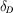and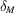, which represent spot diameter and reflecting range, respectively. It is assumed that the coordinates of all image pixels contributing to the intensity of a spot satisfy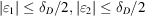and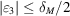when mapped to the profile coordinate system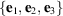defined in Section 11.3.2.3. Regions of neighbouring reflections may overlap. As implemented in XDS, potential overlap is dealt with by a simple strategy: pixels within the overlap region are assigned to the nearest spot. This is carried out in two steps. First, reflections predicted to occur on a given rotation image are found by generating and testing all possible indices h, k, l up to the highest resolution recorded by the detector. Reflection indices, coordinates of the diffracted beam wave vector and the expected fraction of spot intensity recorded on the image are saved in a table. In the second step, each reflection boundary is traced in the image and corrected to exclude pixels belonging to overlapping reflections, which are rapidly located in the table by the hash technique. The image scaling factor obtained from the mean image background and the neighbourhood pixel values belonging to the reflections recorded in the image are saved on a scratch file dedicated to the currently processed data image.

At regular intervals, these files are merged such that all pixel values belonging to a spot found in the contributing images follow each other. Reflections for which contributing pixels are expected further ahead in data processing are just copied to a scratch output file. The other reflections are mapped to the Ewald sphere, as described below, and their three-dimensional profiles and accompanying information are routed to the main output file of the spot-extraction step. After the file-merging procedure, spot extraction continues.

#### 11.3.3.2. Background

| top | pdf |

The region around a spot is assumed to have been chosen to be large enough to include a sufficient number of pixels which can be used for determination of the background. Background determination, as implemented in XDS, begins by sorting all pixels belonging to a reflection by increasing intensity. For weak or absent reflections, these values should represent a random sample drawn from a normal distribution. If this is not the case, the pixel with the largest intensity is removed until the sampling distribution of the remaining smaller items satisfies the expected distribution. This method will also exclude pixels with unexpectedly high values, such as ice reflections. The background, determined as the mean value of the accepted pixels, is systematically overestimated for strong spots because of some residual intensity extending into the accepted background pixels. This residual intensity is estimated from the expected distribution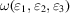defined in Section 11.3.2.3and removed from the final background value.

#### 11.3.3.3. Standard profiles

| top | pdf |

Reflection profiles are represented on the Ewald sphere within a domaincomprising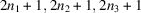equidistant gridpoints along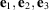, respectively. The sampling distances between adjacent grid points are then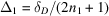,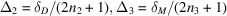. Thus, grid coordinate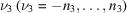covers the set of rotation angles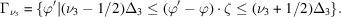Contributions to the spot intensity come from one or several adjacent data images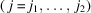, each covering the set of rotation angles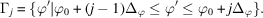Assuming Gaussian profiles along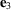for all reflections (see Section 11.3.2.3), the fraction of counts (after subtraction of the background) contributed by data frame j to grid coordinateis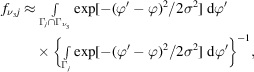where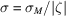. The integrals can be expressed in terms of the error function, for which efficient numerical approximations are available (Abramowitz & Stegun, 1972). Finally, each pixel on data image j belonging to the reflection is subdivided intoareas of equal size, and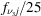of the pixel signal is added to the profile value at grid coordinates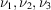corresponding to each subdivision.

This complicated procedure leads to more uniform intensity profiles for all reflections than using their untransformed shape. This simplifies the task of modelling the expected intensity distribution needed for integration by profile fitting. As implemented in XDS, reference profiles are learnt every 5° of crystal rotation at nine positions on the detector, each covering an equal area of the detector face. In the learning phase, profile boxes of the strong reflections are normalized and added to their nearest reference profile boxes. The contributions are weighted according to the distance from the location of the reference profile. Each grid point within the average profile boxes is classified as signal if it is above 2% of the peak maximum. Finally, each profile is scaled such that the sum of its signal pixels normalizes to one. The analytic expressiondefined in Section 11.3.2.3for the expected intensity distribution is only a rough initial approximation which is now replaced by the empirical reference profiles.

#### 11.3.3.4. Intensity estimation

| top | pdf |

If an expected intensity distribution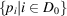of the observed profile is given in a domain, the reflection intensity I can be estimated as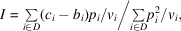which minimizes the function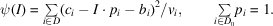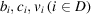are background, contents and variance of pixels observed in a subdomain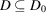of the expected distribution. The backgroundunderneath a diffraction spot is often assumed to be a constant which is estimated from the neighbourhood around the reflection. Determination of reflection intensities by profile fitting has a long tradition (Diamond, 1969; Ford, 1974; Kabsch, 1988b; Otwinowski, 1993). Implementations of the method differ mainly in their assumptions about the variances. Ford uses constant variances, which works well for films, which have a high intrinsic background. In XDS, which was originally designed for a multiwire detector,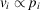was assumed, which results in a straight summation of background-subtracted counts within the expected profile region,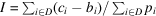. This particular simple formula is very satisfactory for the low background typical of these detectors. For the general case, however, better results can be obtained by using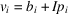for the pixel variances as shown by Otwinowski and implemented in DENZO and in the later version of XDS. Starting with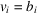, the intensity is now found by an iterative process which is terminated if the new intensity estimate becomes negative or does not change within a small tolerance, which is usually reached after three cycles. It can be shown that the solution thus obtained is unique.

### References

Abramowitz, M. & Stegun, I. A. (1972). Handbook of mathematical functions. New York: Dover Publications.Google Scholar
Diamond, R. (1969). Profile analysis in single crystal diffractometry. Acta Cryst. A25, 43–55.Google Scholar
Ford, G. C. (1974). Intensity determination by profile fitting applied to precession photographs. J. Appl. Cryst. 7, 555–564.Google Scholar
Kabsch, W. (1988b). Evaluation of single-crystal X-ray diffraction data from a position-sensitive detector. J. Appl. Cryst. 21, 916–924.Google Scholar
Otwinowski, Z. (1993). Oscillation data reduction program. In Proceedings of the CCP4 study weekend. Data collection and processing, edited by L. Sawyer, N. Isaacs & S. Bailey, pp. 56–62. Warrington: Daresbury Laboratory.Google Scholar